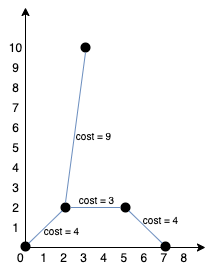# GeetCode Hub

You are given an array `points` representing integer coordinates of some points on a 2D-plane, where `points[i] = [xi, yi]`.

The cost of connecting two points `[xi, yi]` and `[xj, yj]` is the manhattan distance between them: `|xi - xj| + |yi - yj|`, where `|val|` denotes the absolute value of `val`.

Return the minimum cost to make all points connected. All points are connected if there is exactly one simple path between any two points.

Example 1:```Input: points = [[0,0],[2,2],[3,10],[5,2],[7,0]]
Output: 20
Explanation:We can connect the points as shown above to get the minimum cost of 20.
Notice that there is a unique path between every pair of points.
```

Example 2:

```Input: points = [[3,12],[-2,5],[-4,1]]
Output: 18
```

Example 3:

```Input: points = [[0,0],[1,1],[1,0],[-1,1]]
Output: 4
```

Example 4:

```Input: points = [[-1000000,-1000000],[1000000,1000000]]
Output: 4000000
```

Example 5:

```Input: points = [[0,0]]
Output: 0
```

Constraints:

• `1 <= points.length <= 1000`
• `-106 <= xi, yi <= 106`
• All pairs `(xi, yi)` are distinct.

class Solution { public int minCostConnectPoints(int[][] points) { } }